# The Gram-Schmidt Process for Orthonormalizing Vectors

Instructor: Gerald Lemay

Gerald has taught engineering, math and science and has a doctorate in electrical engineering.

Linearly combining things is something we do quite naturally. When the things are vectors, there is a fantastic way to organize the vectors before combining them. In this lesson, we'll show how to orthonormalize vectors using the Gram-Schmidt process.

## The Gram-Schmidt Process

Experimenting with spice mixtures is fun. Let's say our imaginary spices are called altimusX and altimusY, available in premixed formats: satchels of 3 parts altimusX with 4 parts altimusY and satchels of 5 parts altimusY. What if a recipe calls for 1 part altimusX with 4 parts altimusY? How much of each satchel would you combine?

This question leads to the Gram-Schmidt process, which is an algorithm for orthonormalizing vectors. To better appreciate the process, we start with independent vectors and basis vectors. In the recipe analogy, the satchels are like independent vectors. Then we produce orthogonal basis vectors.

## Combining Vectors

Three of altimusX and 4 of altimusY can be written as (3,4), which look like vectors. For example, v1 = (3,4), and v2 = (0,5). Vectors have length and direction; for the purposes of this lesson, we are interested in vectors with different directions. These vectors are independent, like the v1 and v2 vectors shown below: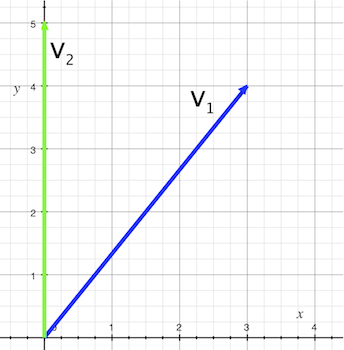Independent vectors can form a basis, meaning other vectors in the same space can be written as a combination of these vectors. In our recipe analogy, we desired a mixture of 1 altimusX with 4 altimusY. Here, d = (1, 4) is a linear combination, or expression, of v1 and v2.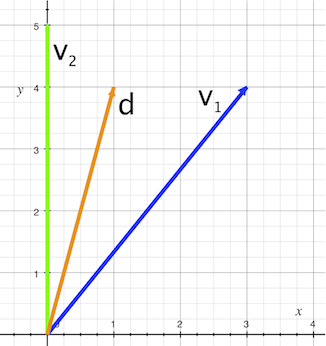Let's perform some calculations:

• 1/3 of v1 + 8/15 of v2 = d
• 1/3 of v1 (1/3 times 3, 1/3 times 4) = (1, 4/3)
• 8/15 of v2 (8/15 times 0, 8/15 times 5) = (0, 8/3)
• 1/3 of v1 + 8/15 of v2 = (1, 4/3) + (0, 8/3)
• (1 + 0, 4/3 + 8/3) = (1, 12/3) = (1,4)

You might be asking how we got the 1/3 and the 8/15: by solving two equations and two unknowns. But there is a better way to have linear combinations because there is a better basis. We could use an orthonormal basis, or one where not only are the basis vectors independent, but also perpendicular to each other (the 'ortho' part of orthonormal). And even better, each vector has a length of 1 (the 'normal' part of orthonormal).

You probably already know the standard orthonormal basis vectors: (1,0) and (0,1) in two dimensions. To make things more interesting, we are going to keep v1 and use it with v2 to get another orthonormal basis.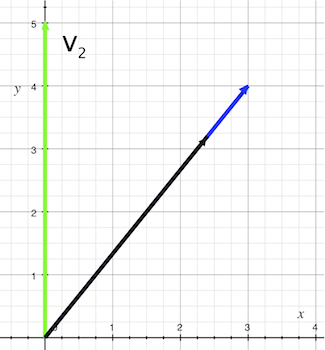To get a vector perpendicular to v1, we project v2 onto v1. This is like the sun casting a shadow of v2 onto v1. See the black vector in the figure? Then, we reverse the direction of the projection: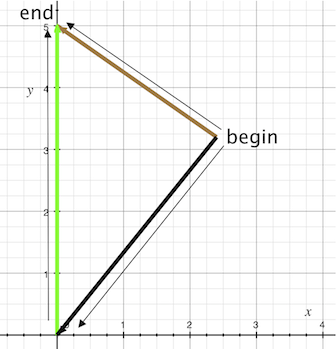Getting from 'begin' to 'end' with the brown vector shown above is the same as adding the reversed projection of v2 (the black vector) with v2 (the green vector). Now, shift the brown vector to the origin without changing its direction or length. The color changes back to green to remind us it comes from the v2 vector.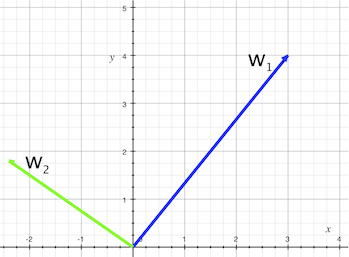Now it's time to change the names of those vectors:

• v1 is now w1
• The new vector perpendicular to v1 is w2

We then divide w1 by its length (written ||w1||) and w2 by its length (written ||w2||. The vectors now have unity in length, and we label them u1 and u2. Their values are:

• u1 = (.6, .8)
• u2 = (-.8, .6)

Now, our desired vector d is a linear combination of the u1 and u2 orthonormal basis vectors, as shown below.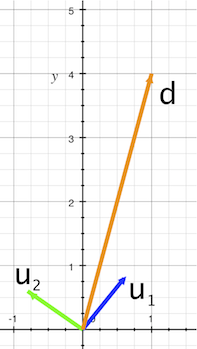We can use the inner product, or the 'dot product', to find the proportions. Let's get right to it by calculating the inner product of v1 with v2:

• v1 · v2
• (3,4) · (0,5)
• 3 times 0 + 4 times 5
• 0 + 20 = 20

How about the inner product of our orthonormal vectors, u1 and u2:

• u1 · u2
• (.6, .8) · (-.8, .6)
• .6 times -.8 + .8 times .6
• -.48 + .48 = 0

The inner product of 'ortho' vectors is 0, but what about the inner product of a vector with itself?

• v1 · v1
• (3,4) · (3,4)
• 3 times 3 + 4 times 4 = 9
• 9 + 16 = 25

The square root of 25 is 5. This is ||v1||. To find the length of u1 and u2:

• Take the inner product, u1· u1
• (.6, .8) · (.6, .8)
• .6 times .6 + .8 times .8 = .36
• 36 + .64 = 1.

The length of u1 is the square root of 1, which is 1. The same is true for u2. To find the portions in the linear combination, we write: d = au1 + bu2.

Taking the inner product with u1 of both sides:

• d · u1
• au1 · u1+ bu2 · u1

As u1 · u1 is 1, and u2 · u1 is 0, d · u1 = a. By evaluating, we see that:

• d · u1
• (1,4) · (.6, .8) = .6
• .6 + 3.2 = 3.8.

What about b and u2? Here:

To unlock this lesson you must be a Study.com Member.

### Register to view this lesson

Are you a student or a teacher?

### Unlock Your Education

#### See for yourself why 30 million people use Study.com

##### Become a Study.com member and start learning now.
Back
What teachers are saying about Study.com

### Earning College Credit

Did you know… We have over 160 college courses that prepare you to earn credit by exam that is accepted by over 1,500 colleges and universities. You can test out of the first two years of college and save thousands off your degree. Anyone can earn credit-by-exam regardless of age or education level.